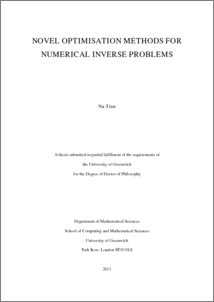# Novel optimisation methods for numerical inverse problems

Tian, Na (2011) Novel optimisation methods for numerical inverse problems. PhD thesis, University of Greenwich.Preview
PDF
Na_Tian_2011.pdf - Published Version

## Abstract

Inverse problems involve the determination of one or more unknown quantities usually appearing in the mathematical formulation of a physical problem. These unknown quantities may be boundary heat flux, various source terms, thermal and material properties, boundary shape and size. Solving inverse problems requires additional information through in-situ data measurements of the field variables of the physical problems. These problems are also ill-posed because the solution itself is sensitive to random errors in the measured input data. Regularisation techniques are usually used in order to deal with the instability of the solution. In the past decades, many methods based on the nonlinear least squares model, both deterministic (CGM) and stochastic (GA, PSO), have been investigated for numerical inverse problems.

The goal of this thesis is to examine and explore new techniques for numerical inverse problems. The background theory of population-based heuristic algorithm known as quantum-behaved particle swarm optimisation (QPSO) is re-visited and examined. To enhance the global search ability of QPSO for complex multi-modal problems, several modifications to QPSO are proposed. These include perturbation operation, Gaussian mutation and ring topology model. Several parameter selection methods for these algorithms are proposed. Benchmark functions were used to test the performance of the modified algorithms. To address the high computational cost of complex engineering optimisation problems, two parallel models of the QPSO (master-slave, static subpopulation) were developed for different distributed systems. A hybrid method, which makes use of deterministic (CGM) and stochastic (QPSO) methods, is proposed to improve the estimated solution and the performance of the algorithms for solving the inverse problems.

Finally, the proposed methods are used to solve typical problems as appeared in many research papers. The numerical results demonstrate the feasibility and efficiency of QPSO and the global search ability and stability of the modified versions of QPSO. Two novel methods of providing initial guess to CGM with approximated data from QPSO are also proposed for use in the hybrid method and were applied to estimate heat fluxes and boundary shapes. The simultaneous estimation of temperature dependent thermal conductivity and heat capacity was addressed by using QPSO with Gaussian mutation. This combination provides a stable algorithm even with noisy measurements. Comparison of the performance between different methods for solving inverse problems is also presented in this thesis.

Item Type: Thesis (PhD) uk.bl.ethos.571431 numerical inverse problems, algorithms, mathematics Q Science > QA Mathematics School of Computing & Mathematical SciencesSchool of Computing & Mathematical Sciences > Department of Mathematical Sciences 13 Apr 2017 16:17 None None None None http://gala.gre.ac.uk/id/eprint/9099View Item